Impact Factor 3.560 | CiteScore 3.1
More on impact ›

# Frontiers in Physics

## PERSPECTIVE article

Front. Phys., 13 June 2014 | https://doi.org/10.3389/fphy.2014.00032

# The Higgs boson and the International Linear Collider

• 1Institute for International Education, Tohoku University, Aoba ku, Sendai, Japan
• 2Physics Department, Tohoku University, Aramaki, Sendai, Japan
• 3Scuola Internazionale Studi Superiori Avanzati, Trieste, Italy

The Higgs boson will be subject of intense experimental searches in future high-energy experiments. In addition to the effort made at the Large Hadron Collider, where it was discovered, it will be the major subject of study at the International Linear Collider. We review here the reasons for that and some of the issues to be tackled at this future accelerator, in particular that of the precision of the Higgs-boson couplings.

The Large Hadron Collider (LHC) at CERN has brought physics to the TeV energy frontier. It has been a long-standing belief that at this energy an important issue regarding the Standard Model (SM) of particle physics would be answered and New Physics (NP) (as in addition to that described by the SM) would be observed.

In its first stage, at 7 and 8 TeV of center of mass (CM) energy, however, no NP has been found, leaving physicists uncomfortably bewildered about their long-held view that TeV- or even subTeV-NP is responsible for the stabilization of the Fermi scale and of the Higgs mass. While the final answer to this issue has still to be given by the second stage of the LHC at CM energy of 14 TeV, at least one part of physicists expectations was realized: in July 2012, the discovery of a new boson was announced [1,2]. In the following months, it became increasingly clear that this particle is indeed the Higgs boson responsible for the breaking of the SM gauge symmetry and for providing mass to the SM elementary particles.

The frantic activity to fingerprint this particle will, without any doubt, increase in the second LHC stage. In case NP turns out to be more elusive than expected, the measurement of the Higgs-boson's couplings can already give us information on what we are to find. Any top-like NP state X capable of stabilizing the Fermi scale and the Higgs mass itself, for example, is bound to produce a deviation of the couplings Higgs-gluon-gluon gHgg and Higgs-photon-photon gHγγ, with resepct to the SM couplings gHgg|SM and gHγγ|SM, which can be numerically expressed as1:

if the state X is a top-like scalar, or

$gHgggHgg|SM=1+2.9% (TeVmX)2, gHγγgHγγ|SM ​=​ 1 ​− ​0.8% (TeVmX)2,(2)$

if it is a top-like fermion. Thus, a resolution of the couplings gHgg and gHγγ at the percent level is needed to tell us something about this possible new state X.

Supersymmetric particles, if Supersymmetry (SUSY) is the theory that extends the SM, produce numerically similar deviations of the gHgg and gHγγ couplings, in the minimal SUSY SM with mA = 1 TeV, tan β = 1, and stop particles with masses 860 and 1200 GeV:

$gHgggHgg|SM=1−2.7, gHγγgHγγ|SM=1+0.2,(3)$

with a peculiar change of signs with respect to those obtained in Equations (1), (2). This is due to the fact that in the SUSY situation charginos/charged Higgs/staus contribute only to the gHγγ coupling, whereas stops contribute to both.

Larger deviations can be obtained in other models, but it is clear that to disentangle at least the simple extensions discussed above, a precision at the percent level, or even smaller, is required. It is then clear why the measurement precision of the Higgs couplings has become an arena for debate on which new accelerators, if any, should guide us into future explorations.

The importance of e+e machines for these future explorations of particle physics has long been discussed (see for example , ).

Lepton e+e colliders have quite some advantage over hadron colliders when it comes to precision measurements.

In a hadron machine, as the LHC, about 30 pp collisions take place at each bunch crossing with each of them producing hundreds of particles. At an e+e collider only one photon-photon collision is expected at each bunch crossing. Thus, detectors for e+e machines are unburdened from high-occupancy problems. They can be as thin as possible and physically located much closer to the interaction point. In turn, this allows a factor of 10 improvement in momentum resolution, with respect to a hadron collider like the LHC, a factor of about three improvement in jet-energy resolution, and, last, but not least, also an excellent capability of tagging b- and c- quarks, as well as τ- leptons.

Together with the fact that pileups from multiple collisions are not a problem in an e+e environment, this makes clear why an e+e machine is more suited for precision measurements.

The unique feature of the International Linear Collider (ILC) is the fact that its CM energy can be increased gradually simply by extending the main linac.

The ILC is supposed to operate at three stages. After a start at $\sqrt{{s}}$ = 250 GeV, there will be an increase of the CM energy to 500 GeV, and later to 1000 GeV. In the three stages, each one operating for 3 years, an integrated luminosity of 250, 500, and 1000 fb−1 will be obtained, respectively. With a luminosity upgrade, the accumulated luminosity will reach values of 1150, 1600, and 2500 fb−1, respectively. Given these luminosities, it is reasonable to assume that at the 250 and 500 GeV ILC, about 80 thousend and 120 thousand Higgs events will be produced. With the luminosity achieved after a luminosity upgrade, this amount will be at least tripled.

At the different stages the Higgs couplings are measured in different processes. The two main Higgs-production mechanisms at the ILC are Higgs-strahlung, i.e., the radiation of the Higgs bosons off an s-channel Z-line, and the WW-fusion process. The two mechanisms are depicted diagrammatically in Figure 1. A replacement of the W boson with the Z boson and of the neutrino and antineutrino with an electron and a positron in the diagram for the WW-fusion mechanism is the diagram for the sub-leading ZZ-fusion production mechanism.

FIGURE 1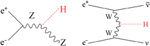Figure 1. Feynman diagrams for the dominant Higgs-production processes at the ILC: the Higgs-strahlung (left) and the WW-fusion processes (right).

As shown by the values of cross sections for the first two production mechanisms, in Figure 2, the Higgs-strahlung process peaks at $\sqrt{{s}}$ = 250 GeV, while at higher energies, the dominant production process is the WW-fusion. The blue line for the WW-fusion cross section in this figures includes also the cross section for the ZZ-fusion process, which is only a tiny fraction of that for the WW-fusion process.

FIGURE 2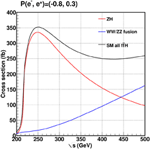Figure 2. Cross sections for the Higgs-strahlung (red line) and WW-fusion (blue line) production mechanism, as a function of the CM energy $\sqrt{{s}}$ . The black line is simply the cross section due to both production mechanisms, giving rise to the final state Hff (with the Z-boson in the Higgs-strahlung cross section decaying in all possible fermions). Polarizations of 80 and 30% for electrons and positrons, respectively, were assumed.

With the subsequent decays of the Higgs boson into a pair of X particles, HXX, these two processes allow to study the couplings gHXX, which can be tree-level couplings, or also effective couplings for loop-level decays. Indeed, the particle X here can be a fermion, a photon, or a gluon.

The ZH process is crucial for measuring the Higgs couplings. By reconstructing the Z boson from the two leptons to which it decays, the Higgs boson can be captured inclusively, without looking at its decay products. Thus, also the width for invisible decays can be estimated.

With the Higgs recoil method, and the luminosity values specified above, the total cross section for the ZH process, σZH, can be measured with 1.3% precision.

Measuring then the cross section for the process e+eZHZXX, σZH;HXX, sensitive to the coupling gHXX:

$σZH;H→XXσZH∝gHXX2(4)$

a good precision can be achieved for the couplings gHXX. In particular a precision of 0.7% can be obtained in the case X = Z, i.e., for gHZZ, when mH = 126 GeV is used. (Note the claimed precision of 0.5% listed in Table 1, referring to mH = 120 GeV.

TABLE 1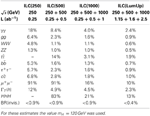Table 1. Expected precisions for the Higgs-boson couplings , once results from the different CM energies, and upgraded luminosity are considered.

The most challenging decays among those studied at this CM energy, are those into b-, c- quarks, and into gluons.

The expected sensitivity for the couplings measured at this stage is summarized in Table 1, where also the couplings gHWW, gHtt, measured at 500 GeV, and discussed hereafter are included.

At $\sqrt{{s}}$ = 500 GeV, the measurement of the cross section for the process e+e → ννH → ννWW, σννH;HWW, related to gHWW as in

$σνν¯H;H→WW=σνν¯HBR(H→WW)∝gHWW4(5)$

gives this coupling, up to the absolute cross section σννH. This can be obtained from the measurement of σννH;HXX:

$σνν¯H;H→XX=σνν¯HBR(H→XX)∝gHWW2gHXX2(6)$

with the coupling g2HXX already extracted at $\sqrt{{s}}$ = 250 GeV. A convenient decay mode is in this case Hbb.

At $\sqrt{{s}}$ = 500 GeV also the ttH-production mechanism opens up. The relevant Feynman diagrams for this process are shown in Figure 3.

FIGURE 3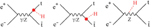Figure 3. Feynman diagrams for the ttH production process, with the Higgs boson radiated off the t-quark and the Z-line.

The corresponding cross section is plotted in Figure 4 as a function of the CM energy. At $\sqrt{{s}}$ = 500 GeV the cross section is somewhat small. It is however enhanced by a factor of two by QCD corrections, including mainly tt bound-state effects. This makes the measurement of the Higgs-top coupling possible, with a somewhat modest precision of 14%, not including theoretical errors. Subsequent CM-energy increases and luminosity upgrades will be able to improve this precision up to 2% (again without theory errors).

FIGURE 4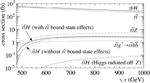Figure 4. Cross section for the ttH process as a function of $\sqrt{{s}}$ . The solid(dotted) lines, highlighted by arrows, denote which one includes(doesn't include) QCD effects. Both lines refer to the process shown in Figure 3. For completeness it is shown also the ttH process originating from the Higgs strahlung, with subsequent decay Htt. Shown are also some sources of background, such as the process ttg* ≥ ttbb with a virtual gluon radiated off one of the two initial top-quarks.

Data at 500 and 1000 GeV will help to improve the precision of all couplings already measured in previous stages.

What will remain a bit elusive, probably until the completion of the ILC program, is the Higgs self coupling, gHHH. It is clear that to give a complete identification of the recently discovered Higgs boson the measurement of this self coupling and then a reconstruction of the Higgs potential are necessary.

It can be obtained from the ZH and WW-fusion processes, with a subsequent decay of H into a pair HH. In the e+eZHH process the Z boson will be reconstructed from the pairs ll and qq in which it decays. In the e+e → ννHH the two H's can be reconstructed from 4b's or 2b's and 2 W's.

Luminosity upgrade at the ILC as well as high polarization will have the goal of bringing the measurement of the Higgs self coupling to a final ~10% precision.

Except for this coupling, the precision achievable for the other couplings, summarized in Table 1, is well within the values requested by Equations (1, 2, 3), i.e., is good enough to address the issue of the nature of NP to be expected.

The LHC will in the meantime help (hopefully even considerably) in fingerprinting the Higgs boson. Together with the ILC, then, an accurate profile of this particle will be put together and, with it, an understanding of what triggers the electroweak symmetry breaking will be obtained.

## Conflict of Interest Statement

The authors declare that the research was conducted in the absence of any commercial or financial relationships that could be construed as a potential conflict of interest.

## Acknowledgments

The work of Francesca Borzumati is partially supported by the Grant-in-Aid for Scientific Research 23540283 of JSPS, Japan, and the ERC Advanced Grant no. 267985 “DaMESyFla.” Eriko Kato is supported by the JSPS Grant in Aid for Specially-Promoted Research “A global R&D program of a state-of-the-art detector system for ILC.”

## Footnotes

1. ^All the following estimates are from Baer et al. , where the value of 120 GeV was chosen for the Higgs-boson mass. An update of all previous studies, using mH = 126 GeV is still missing.

## References

1. Aad G, Abajyan T, Abbott B, Abdallah J, Abdel Khalek S, Abdelalim AA, et al. [ATLAS Collaboration]. Observation of a new particle in the search for the Standard Model Higgs boson with the ATLAS detector at the LHC, [ATLAS Collaboration]. Phys Lett. (2012) B716:1. arXiv:1207.7214 [hep-ex]

2. Chatrchyan S, Khachatryan V, Sirunyan AM, Tumasyan A, Adam W, Aguilo E, et al. [CMS Collaboration]. Observation of a new boson at a mass of 125 GeV with the CMS experiment at the LHC, [CMS Collaboration]. Phys Lett. (2012) B716:30. arXiv:1207.7235 [hep-ex]

3. Baer H, Barklow T, Fujii K, Gao Y, Hoang A, Kanemura S, et al. The International Linear Collider Technical Design Report, Physics (2013) Vol. 2. arXiv:1306.6352 [hep-ph]

4. Kato E. LC13: Exploring QCD From The Infrared Regime to Heavy-Flavor Scales at B Factories, The LHC And A Linear Collider, ECT*, Contribution to The Workshop. Nuov Cim. C037N2. (2014) 37:4. Trento, Italy.

5. Accomando E, Andreazza A, Anlauf H, Ballestrero A, Barklow T, Bartels J, et al. [ECFA/DESY LC Physics Working Group Collaboration]. Physics with e+ e linear colliders, [ECFA/DESY LC physics working group collaboration]. Phys Rept. (1998) 299:1. [hep-ph/9705442]

6. Asner DM, Barklow T, Calancha C, Fujii K, Graf N, Haber HE, et al. ILC Higgs White Paper. High Energy Physics - Phenomenology (2013) arXiv:1310.0763 [hep-ph]

7. Yonamine R, Ikematsu K, Tanabe T, Fujii K, Kiyo Y, Sumino Y, et al. Measuring the top Yukawa coupling at the ILC at $\sqrt{{s}}$ = 500 GeV. Phys Rev. (2011) D84:14033. arXiv:1104.5132 [hep-ph]

Keywords: particle physics phenomenology, physics beyond the Standard Model, Higgs production, Higgs decays, International Linear Collider

Citation: Borzumati F and Kato E (2014) The Higgs boson and the International Linear Collider. Front. Physics 2:32. doi: 10.3389/fphy.2014.00032

Received: 25 February 2014; Accepted: 08 May 2014;
Published online: 13 June 2014.

Edited by:

Stefano Moretti, University of Southampton, UK

Reviewed by:

Abdesslam Arhrib, University AbdelMalek Essaadi, Morocco
Rikard Enberg, Uppsala University, Sweden

Copyright © 2014 Borzumati and Kato. This is an open-access article distributed under the terms of the Creative Commons Attribution License (CC BY). The use, distribution or reproduction in other forums is permitted, provided the original author(s) or licensor are credited and that the original publication in this journal is cited, in accordance with accepted academic practice. No use, distribution or reproduction is permitted which does not comply with these terms.

*Correspondence: Francesca Borzumati, Scuola Internazionale Studi Superiori Avanzati, Via Bonomea, 265 - 34136 Trieste, Italy e-mail: fborzumati@gmail.com